# Coordinate Geometry Class 10 Notes

## Class 10 Maths Chapter 7 Coordinate Geometry Notes

The complete notes on coordinate geometry for Class 10 are provided here. Coordinate geometry teaches us the location of a point on a plane. For example, the coordinates of a point are (x, y), where x-coordinate (abscissa) denotes the distance of a point from the y-axis and y-coordinate (ordinate) denotes the distance of the point from the x-axis. Now we will learn to find the distance between two points and the area of a triangle using the distance formula when the coordinates of the points are given. Go through the below article and learn the points on the coordinate plane, distance formulas, section formulas and so on with a detailed explanation.

Students can refer to the short notes and MCQ questions along with a separate solution pdf of this chapter for quick revision from the links below:

## Basics of Coordinate Geometry

#### For more information on the Basics of Coordinate Geometry, watch the below videoTo know more about Coordinate Geometry, visit here.

### Points on a Cartesian Plane

A pair of numbers locate points on a plane called the coordinates. The distance of a point from the y-axis is known as abscissa or x-coordinate. The distance of a point from the x-axis is called ordinate or y-coordinate.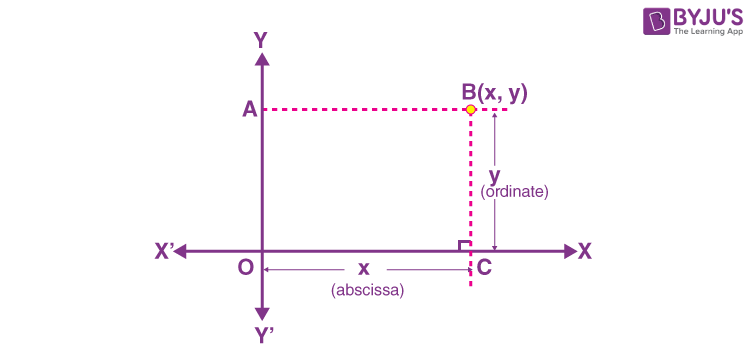Example: Consider a point P(3, 2), where 3 is the abscissa, and 2 is the ordinate. 3 represents the distance of point P from the y-axis, and 2 represents the distance of point P from the x-axis.

## Distance Formula

### Distance between Two Points on the Same Coordinate Axes

The distance between two points that are on the same axis (x-axis or y-axis) is given by the difference between their ordinates if they are on the y-axis, else by the difference between their abscissa if they are on the x-axis.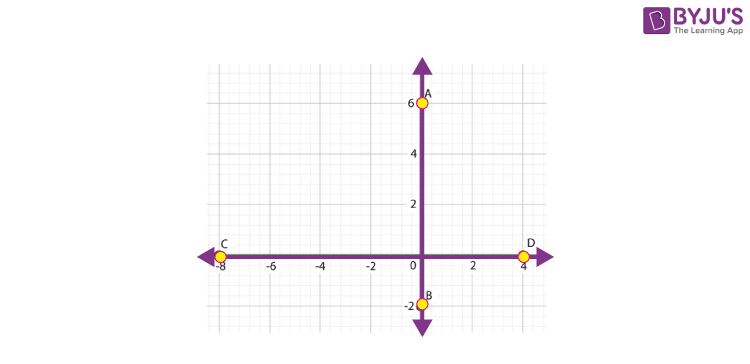Distance AB = 6 – (-2) = 8 units

Distance CD = 4 – (-8) = 12 units

### Distance between Two Points Using Pythagoras Theorem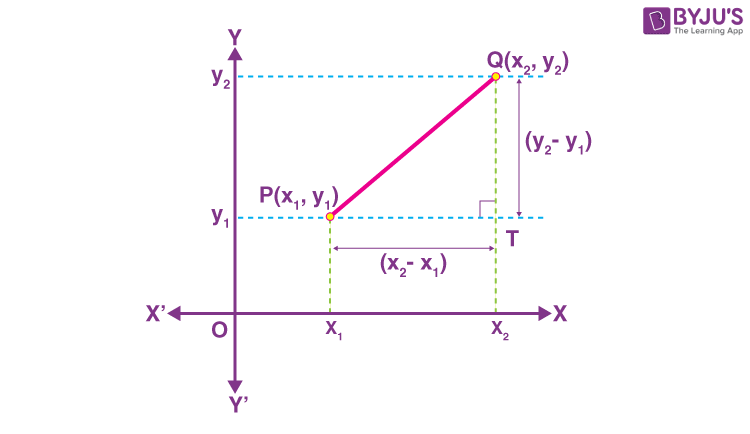Let P(x1, y1) and Q(x2, y2) be any two points on the cartesian plane.

Draw lines parallel to the axes through P and Q to meet at T.

ΔPTQ is right-angled at T.

By Pythagoras Theorem,

PQ2 = PT2 + QT2

= (x2 – x1)+ (y2 – y1)2

PQ = √[x2 – x1)+ (y2 – y1)2]

### Distance Formula

Distance between any two points (x1, y1) and (x2, y2) is given by

d = √[x2 – x1)2+(y2 – y1)2]

Where d is the distance between the points (x1,y1) and (x2,y2).

To know more about Distance Formula, visit here.

## Section Formula

If the point P(x, y) divides the line segment joining A(x1, y1) and B(x2, y2) internally in the ratio m:n, then, the coordinates of P are given by the section formula as:

$$\begin{array}{l}P(x,\ y)=(\frac{mx_{2}+nx_{1}}{m+n},\frac{my_{2}+ny_{1}}{m+n} )\end{array}$$To know more about Section Formula, visit here.

### Finding Ratio given the points

To find the ratio in which a given point P(x, y) divides the line segment joining A(x1, y1) and B(x2, y2),

• Assume that the ratio is k : 1
• Substitute the ratio in the section formula for any of the coordinates to get the value of k.
$$\begin{array}{l}x=\frac{kx_{2}+x_{1}}{k+1}\end{array}$$

When x1, x2 and x are known, k can be calculated. The same can be calculated from the y- coordinate also.

Example: Find the ratio when point (– 4, 6) divide the line segment joining the points A(– 6, 10) and B(3, – 8)?

Solution: Let the ratio be m:n.

We can write the ratio as:

m/n : 1 or k:1

Suppose (-4, 6) divide the line segment AB in k:1 ratio.

Now using the section formula, we have the following;

$$\begin{array}{l}(-4, 6) = (\frac{3k-6}{k+1},\frac{-8k+10}{k+1})\end{array}$$

-4 = (3k-6)/(k+1)

– 4k – 4 = 3k – 6

7k =2

k : 1 = 2 : 7

Thus, the required ratio is 2:7.

### Midpoint

The midpoint of any line segment divides it in the ratio 1 : 1.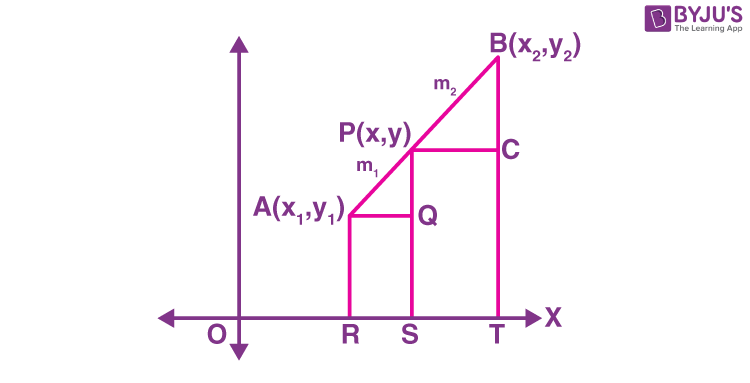The coordinates of the midpoint(P) of line segment joining A(x1, y1) and B(x2, y2) is given by

$$\begin{array}{l}p(x,\ y)=(\frac{x_{1}+x_{2}}{2},\frac{y_{1}+y_{2}}{2} )\end{array}$$

Example: What is the midpoint of line segment PQ whose coordinates are P (-3, 3) and Q (1, 4), respectively.

Solution: Given,  P (-3, 3) and Q (1, 4) are the points of line segment PQ.

Using midpoint formula, we have;

$$\begin{array}{l}Midpoint ~of~ PQ = (\frac{-3+1}{2}, \frac{-3+4}{2})\end{array}$$

= (-2/2, 1/2)

= (-1, 1/2)

### Points of Trisection

To find the points of trisection P and Q, which divides the line segment joining A(x1, y1) and B(x2, y2) into three equal parts:

i) AP : PB = 1 : 2

$$\begin{array}{l}P=(\frac{x_{2}+2x_{1}}{3},\frac{y_{2}+2y_{1}}{3} )\end{array}$$

ii) AQ : QB = 2 : 1

$$\begin{array}{l}Q=(\frac{2x_{2}+x_{1}}{3},\frac{2y_{2}+y_{1}}{3} )\end{array}$$

Example: Find the coordinates of the points of trisection of the line segment joining the points A(2, – 2) and B(– 7, 4).

Solution: Let P and Q divide the line segment AB into three parts.

So, P and Q are the points of trisection here.

Let P divides AB in 1:2, thus by section formula, the coordinates of P are (1, 0)

Let Q divides AB in 2:1 ratio, then by section formula, the coordinates are (-4,2)

Thus, the point of trisection for line segment AB are (1,0) and (-4,2).

### Centroid of a Triangle

If A(x1, y1), B(x2, y2), and C(x3, y3) are the vertices of a ΔABC, then the coordinates of its centroid(P) are given by

$$\begin{array}{l}p(x,\ y)=(\frac{x_{1}+x_{2}+x_{3}}{3},\frac{y_{1}+y_{2}+y_{3}}{3} )\end{array}$$

Example: Find the coordinates of the centroid of a triangle whose vertices are given as (-1, -3), (2, 1) and (8, -4)

Solution: Given,

The coordinates of the vertices of a triangle are (-1, -3), (2, 1) and (8, -4)

The Centroid of a triangle is given by:

G = ((x1+x2+x3)/3 , (y1+y2+y3)/3 )

G = ((-1+2+8)/3 , (-3+1-4)/3)

G =( 9/3 , -6/3)

G = (3, -2)

Therefore, the centroid of a triangle, G = (3, -2)

## Area from Coordinates

### Area of a Triangle given its vertices

If A(x1, y1),B(x2, y2) and C(x3, y3) are the vertices of a Δ ABC, then its area is given by

A = (1/2)[x1(y2 − y3) + x2(y3 − y1) + x3(y1 − y2)]

Where A is the area of the Δ ABC.

Example: Find the area of the triangle ABC whose vertices are A(1, 2), B(4, 2) and C(3, 5).

Solution:

Using the formula given above,

A = (1/2) [x1 (y2 – y3 ) + x2 (y3 – y1 ) + x3(y1 – y2)]

A = (1/2) [1(2 – 5) + 4(5 – 2) + 3(2 – 2)]

A = (1/2) [-3 + 12]

Area = 9/2 square units.

Therefore, the area of a triangle ABC is 9/2 square units.

To know more about the Area of a Triangle, visit here.

### Collinearity Condition

If three points A, B and C are collinear and B lies between A and C, then,

• AB + BC = AC. AB, BC, and AC can be calculated using the distance formula.
• The ratio in which B divides AC, calculated using the section formula for both the x and y coordinates separately, will be equal.
• The area of a triangle formed by three collinear points is zero.

## Video Lesson on Coordinate Geometry Toughest Problems### Coordinate Geometry for Class 10 Problems

Example 1:

Determine the distance between the pair of points (a, b) and (-a, -b)

Solution:

Let the given points be A(a, b) and B(-a, -b)

We know that the distance formula is:

AB = √[(x2-x1)2+(y2-y1)2]

(x1, y1) = (a, b)

(x2, y2) = (-a, -b)

Now, substitute the values in the distance formula, we get

AB = √[(-a-a)2+(-b-b)2]

AB = √[(-2a)2 + (-2b)2]

AB = √[4a2+4b2]

AB = √[4(a2+b2)]

AB = √4. √[a2+b2]

AB = 2.√[a2+b2].

Hence, the distance between two points (a, b) and (-a, -b) is 2√[a2+b2].

Example 2:

Determine the ratio in which the line segment joining the points A(1, -5) and B(-4, 5) is divided by the x-axis. Also, find the coordinates of the point of division.

Solution:

Given that, the point P is on the x-axis. Hence, the y-coordinate is 0. Hence, the point is of the form P(x, 0).

Now, we have to find the ratio. Let the ratio be k:1.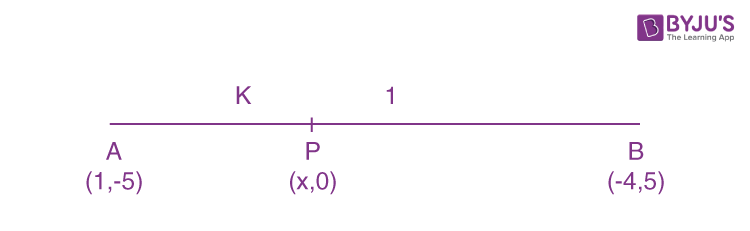Given Points: A(1, -5) and B = (-4, 5).

(x1, y1) = (1, -5)

(x2, y2) = (-4, 5)

m1 = k, m2 = 1

We know that the section formula is:

y= [m1y2+m2y1]/[m1+m2]

Now, substitute the values in the section formula, we get

y = [k(5) + 1(-5)]/[k+1]

y = [5k-5]/[k+1]

Since, y=0

(5k-5)/(k+1) = 0

5k -5 = 0

5k = 5

k=1

Hence, the ratio k:1 = 1:1

Finding x-coordinate:

x= [m1x2+m2x1]/[m1+m2]

x = [k(-4) + 1(1)]/(k+1)

Now, substitute k=1 in the above equation, we get

x = [1(-4) + 1(1)]/(1+1)

x = (-4+1)/2

x = -3/2.

Hence, the coordinate of the point is P(x, 0) = P(-3/2, 0).

## Related Articles

Stay tuned with BYJU’S – The Learning App and download the app to learn all Maths-related concepts easily by exploring more videos.

Test your knowledge on Coordinate Geometry For Class 10